# SSAT Upper Level Math : How to find slope

## Example Questions

2 Next →

### Example Question #11 : Slope

Consider the line of the equation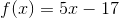. The line of a function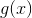has the same slope as that of. Which of the following could be the definition of?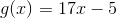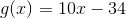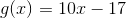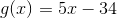The definition ofis written in slope-intercept form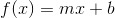, in which, the coefficient of, is the slope of its line., so the slope of its line is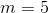.
We must select the choice whose line has this slope. The definition ofin each choice is also written in slope-intercept form, so we select the alternative with-coefficient 5; the only such alternative is.